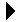Linked Stack Package Body# Linked Stack Package BodyLinked Stack Package Specification Download Linked Stack Client```--
-- This is the body of the linked stack package.
--

-- We'll take care of de-allocation ourselves.  Note that this is a good
-- use of unchecked deallocation, since it is limited to the body of one
-- small package.  That makes it easier to get right.

package body L_Stack is
-- Here's the deallocation function for nodes.
procedure Free is new Unchecked_Deallocation(Stack_Node, Node_Ptr);

-- Push onto the stack.
procedure Push(S: in out Int_Stack; I: Integer) is
begin
S := new Stack_Node'(I, Node_Ptr(S));
end Push;

-- Remove from the stack.
procedure Pop(S: in out Int_Stack; I: out Integer) is
Old_Top: Node_Ptr;
begin
if S /= null then
-- Unlink the top node.
Old_Top := Node_Ptr(S);
S := Int_Stack(S.Next);

-- Get the data out of it.
I := Old_Top.Data;

-- Say bye, little node.
Free(Old_Top);
end if;
end Pop;

-- Find out what's on the top.
function Top(S: Int_Stack) return Integer is
begin
if S = null then
return 0;
else
return S.Data;
end if;
end Top;

-- So, are we empty yet?
function Empty(S: Int_Stack) return Boolean is
begin
return S = null;
end Empty;

-- Kill that stack!
procedure Clean(S: in out Int_Stack) is
Zombie,                   -- Ptr to node about to be deleted.
Scan: Node_Ptr;         -- List scanner.
begin
Scan := Node_Ptr(S);
while Scan /= null loop
-- Advance the pointer and delete the old node.
Zombie := Scan;
Scan := Scan.Next;
Free(Zombie);
end loop;
end Clean;

-- See if two stacks are equal.
function "=" (S, T: Int_Stack) return Boolean is
SScan, TScan: Node_Ptr;  -- List scanners.
begin
-- Init scanners.
SScan := Node_Ptr(S);
TScan := Node_Ptr(T);

-- See if the stacks are equal.
loop
-- If we reach the end of both lists at the same time without
-- a previous return, they must have been equal (found no
-- differences).
if SScan = null and TScan = null then
return True;
end if;

-- If we reach the end of one without the other, the lists are of
-- different lengths.
if SScan = null or TScan = null then
return False;
end if;

-- If the current item disagrees, then not equal.
if SScan.Data /= TScan.Data then
return False;
end if;

-- Next item.
SScan := SScan.Next;
TScan := TScan.Next;
end loop;
end "=";

end L_Stack;
```Linked Stack Package Specification Linked Stack Client